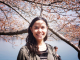Gianna Cifredo
15

15 Hardest SAT Math Questions

Is your SAT score enough to get you into your dream school?

Our free chancing engine takes into consideration your SAT score, in addition to other profile factors, such as GPA and extracurriculars. Create a free account to discover your chances at hundreds of different schools.

Students often want to prepare for the toughest questions they’ll see on the SAT so they can feel confident on test day. We’ve rounded up some of the hardest questions and we’re going to show you how to solve them.

These questions come from the free SAT practice tests because we wanted to make sure that we show you questions representative of those you’ll see on the real test. We’ve chosen a variety of question types, but this isn’t an exhaustive representation of all the math topics or types of questions on the SAT.

“Hard” is a little subjective; we think these questions are difficult based on working with many students, but you may find some of them easy. In general, you can expect to find harder questions in the second half of each SAT math section, as the questions generally increase in difficulty.

For each question below, we’ve identified the key concepts being tested and whether a calculator is allowed. We suggest you try solving these on your own before looking at the answer and our suggested solution. Remember: we’ve given you just one way to solve the problem. Most problems can be solved in a variety of ways!

Math Topics on the SAT

Before we go into the questions, we want you to understand the terms that the College Board uses to categorize the topics. We’ve also included how many questions fall under each category, so if you’re self-studying, you can prioritize the types of questions that appear more often.

Heart of Algebra: 33% of test, 19 questions

Linear equations and inequalities and their graphs and systems.

Problem Solving and Data Analysis: 29% of test, 17 questions

Ratios, proportions, percentages, and units; analyzing graphical data, probabilities, and statistics.

Passport to Advanced Math: 28% of test, 16 questions

Identifying and creating equivalent expressions; quadratic and nonlinear equations/functions and their graphs.

Additional Topics in Math: 10% of test, 6 questions total

This is the only category without a corresponding subscore, but it has a wide variety of topics, including geometry, trigonometry, radians and the unit circle, and complex numbers.

Grid-in Questions

A grid-in question can test any of the topics above and is found at the end of each portion of the math test, both no-calculator and calculator. Most students find grid-in questions harder than the multiple-choice because some test tactics—like substituting answer choices into the problem—don’t work. You’ll need to find the correct solution without any help from answer choices. If you develop your math skills, however, then these questions won’t be that much more difficult for you.

Now grab a calculator, a pencil and a piece of paper, and let’s review the hardest SAT math questions!

Question 1: Calculator permitted, grid-in response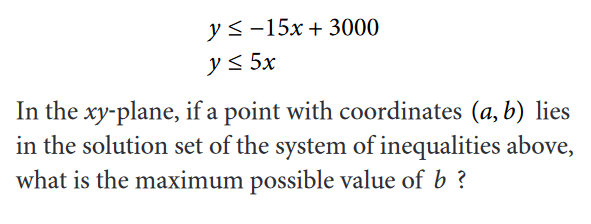Category: Heart of Algebra—systems of linear inequalities

Here’s how to solve it:

1. In this question, we’re told coordinates $$(a,b)$$ lies in the solution set of these equations, and we want to know a maximum possible value. With inequalities, the graph will be shaded to include the set of values that satisfy the inequality, so $$(a,b)$$ lies in this overlapping region. Because it’s a grid-in response (only real numbers can be the answer to the grid-in questions—there is no option to bubble in infinity) we know that the value of $$b$$ must be limited by the point of intersection. Since the inequalities are both less than or equal to, we know that the lines (and the point of intersection) are included in the solution set. If there were only less/greater than symbols, the lines would not be included in the solution set.

1. This means we can find the point of intersection to find a maximum value of $$b$$ and solve it just like a system of equations using the substitution method. This gives us the inequality $$5x\:\leq\:-15x+3000$$. In this case, $$x$$ is equivalent to $$a$$, and the y-value is equivalent to $$b$$.

1. Move the variables to the same side by adding $$15x$$ to both sides. $$20x\leq3000$$.

1. Divide both sides by $$20$$ to find out what $$x$$ is. $$x\:\leq\:150$$. Many students might stop here, but remember we want coordinate $$b$$ in $$(a,b)$$, or the y-value.

1. We can plug $$x$$ into either equation to find the value of $$b$$. We picked the second one since it’s simpler: $$5\:\cdot\:150=750$$. That’s our answer!

Question 2: Calculator permitted, multiple choiceCategory: Problem-solving and data analysis—analyzing graphical data, probability

Here’s how to solve it:

1. With problems like these, it’s important that you pay attention to the wording of the question so that you know exactly what you’re being asked. Once you understand that, the math needed is not necessarily complex. The question asks: “If a person is chosen at random from those who recalled at least 1 dream, what is the probability that the person belonged to Group Y?” It can help to rewrite the question in your own words. Here’s a possible rewrite: “Out of all the people who remembered at least 1 dream, what fraction were in Group Y?”

1. We need to know the total number of people who remembered at least one dream—to do this, we add $$28+57+11+68=164$$.

1. Next, we need to know how many of the people who remembered at least one dream were in Group Y. Add $$11+68=79$$.

1. We can now see that the chance of selecting a person from Group Y out of all the people who remembered at least $$1$$ dream is $$79$$ out of $$164$$, or $$\frac{79}{164}$$, which is C.

Question 3: Calculator not permitted, multiple choice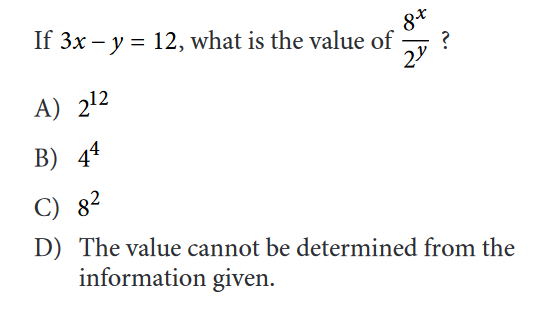Here’s how to solve it:

1. To solve this problem, you’ll need to know exponent rules. Here are some of the ones you should know for the SAT and many other standardized tests:
• $$b^mb^n=b^{m+n}$$
• $$\frac{b^m}{b^n}=b^{m-n}$$
• $$(b^m)^n=b^{m\:\cdot\:n}$$
• $$b^mc^m=(bc)^m$$
• $$\frac{b^m}{c^m}=(\frac{b}{c})^m$$

1. Since we know that it’s likely that we’ll need exponent rules, we can see if we might be able to rewrite the expression $$\frac{8^x}{2^y}$$ to fit one of the existing exponent rules. In fact, we can rewrite $$8=2^3$$, so we have $$8^x=(2^3)^x=2^{3x}$$.

1. Substituting $$2^{3x}$$ for $$8^x$$, we can see that the expression can be rewritten using the second rule above: $$\frac{2^{3x}}{2^y}=2^{3x-y}$$.

1. Since we’ve been given the information that $$3x-y=12$$ we can substitute $$12$$ into the expression and get our answer: $$2^{3x-y}=2^{12}$$.

Question 4: Calculator not-permitted, multiple choiceCategory: Passport to Advanced Math—rational equations

Here’s how to solve it:

1. Students are sometimes intimidated by large fractions like this, but if you get comfortable manipulating fractions and variables across operations then you can handle any question like this. To start, we’ll want to add those two fractions in the denominator, and to do that, we need to find a common denominator. The common denominator in this case is $$(x+2)(x+3)$$.

1. To convert each of those fractions in the denominator to ones we can easily add, we need to find equivalent fractions. You can multiply any fraction by $$1$$ without changing its value, and so we can use fractions like $$\frac{2}{2}$$, etc. We’re going to multiply the first fraction by $$\frac{x+3}{x+3}$$ and the second fraction by $$\frac{x+2}{x+2}$$. This gives us the following in the denominator: $$\frac{x+3}{(x+2)(x+3)}+\frac{x+2}{(x+2)(x+3)}$$

1. Now we can add the numerators: $$(x+3)+(x+2)$$. Combine like terms in the numerator to get $$2x+5$$.

1. We now have a single fraction in the denominator, and we can proceed with fraction division. When dividing by a fraction, we multiply by its reciprocal, and we can find the reciprocal by switching the positions of the numerator and the denominator. We get:

$$\frac{1}{\frac{2x+5}{(x+2)(x+3)}}=1\:\cdot\:\frac{(x+2)(x+3)}{2x+5}=\frac{(x+2)(x+3)}{2x+5}$$

1. Multiplying the numerator out, we can rewrite the expression as $$(x+2)(x+3)=x^2+5x+6$$. This gives us our answer: $$\frac{x^2+5x+6}{2x+5}$$

Question 5: Calculator permitted, grid-in response (see note)Note: This is a two-part question, but we left out the first part to focus on the second one. The first question asked for the value of $$x$$ in the expression. Because her bank account earns $$2\%$$ interest compounded annually, we can convert $$2\%$$ to a decimal, giving us $$.02$$. We then add $$1$$ to this so that we don’t lose the initial deposit. The answer is $$x=1.02$$, which you need to know to solve the question above.

Category: Passport to Advanced Math—exponential functions and equations (interest)

Here’s how you solve it:

1. Given that Jessica uses the expression $$\100(1.02)^t$$ to find the value of her account after $$t$$ years, we can create a similar expression to model Tyshaun’s account. This will be $$\100(1.025)^t$$.

1. Now we can plug $$10$$ in for $$t$$ into both of the expressions. Jessica’s account will have $$\100(1.02)^{10}\approx\121.899$$. Tyshaun’s account will have $$\100(1.025)^{10}\approx\128.008$$.

1. Now that we know how much money each account will have in 10 years, we can subtract the amount of money that Jessica’s account has from the amount of money that Tyshaun’s account has to find our answer. $$\128.008-\121.899=\6.109$$. Pay close attention to how problems ask for the answer: we need to round to the nearest cent and ignore the dollar sign, so the answer is $$6.11$$.

Is your SAT score enough to get accepted to your dream school? Find out with CollegeVine’s free chancing calculator.

Question 6: Calculator not-permitted, multiple choice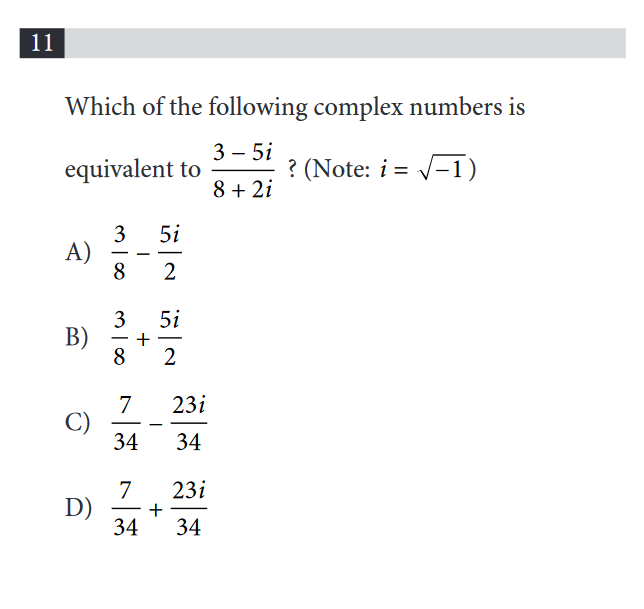Here’s how you solve it:

1. There’s usually at least one question on the SAT involving complex numbers, so it’s helpful to know how to add, subtract, and multiply them together. A complex number in standard form is written $$a+bi$$, where $$a$$ is a real number and $$bi$$ is the imaginary part. In this question, we’ll want to use the conjugate form of $$a+bi$$ which is $$a-bi$$. This is because when we multiply complex conjugates, we get a real number: $$(a+bi)(a-bi)=a^2-abi+abi-b^2i^2=a^2-b^2i^2$$. However, because $$i=\sqrt{-1}$$, that means that $$i^2=-1$$. We can thus simplify $$a^2-b^2i^2=a^2+b^2$$.

1. Taking a hint from the answer choices, which only have real numbers in the first term, we can multiply the fraction above by the conjugate of the denominator like so:

$$\frac{3-5i}{8+2i}\cdot\frac{8-2i}{8-2i}=\frac{(3-5i)(8-2i)}{(8+2i)(8-2i)}$$

1. We can quickly figure out the denominator given the information about conjugates above. $$8^2+2^2=64+4=68$$.

1. Multiplying complex numbers is just like multiplying binomials, keeping in mind that $$i^2=-1$$ and we’ll need to simplify that out at the end.

$$(3-5i)(8-2i)=24-6i-40i+10i^2=24-46i-10=14-46i$$.

1. Our new fraction is this: $$\frac{14-46i}{68}$$. We can rewrite it so that the real part and the imaginary part of the complex numerator are separated and then simplify to get our answer.

$$\frac{14-46i}{68}=\frac{14}{68}-\frac{46i}{68}=\frac{7}{34}-\frac{23i}{34}$$

Question 7: Calculator permitted, multiple choiceCategory: Additional Topics in Math—Circles and their equations

Here’s how you solve it:

1. Like complex numbers, there’s usually at least one question involved the equation of a circle. This equation is not in the standard form of the equation of a circle, which is $$(x-h)^2+(y-k)^2=r^2$$, where $$(h,k)$$ is the center of the circle and $$r$$ is the radius. We’ll need to rewrite this equation into standard form to easily find the radius.

1. To begin rewriting the equation, we will need to complete the square twice, once for $$x$$ and once for $$y$$.

$$x^2+4x+y^2-2y=-1$$

$$(x+2)^2-4+(y-1)^2-1= -1$$

1. As you can see, we halved the absolute value of the $$x$$ and $$y$$ coefficients to find the constant to complete the square, then subtracted the squared constants to keep the equation the same. Now we need to move our constants to the other side by adding and we’ll have our equation in standard form.

$$(x+2)^2+(y-1)^2=-1+1+4$$

$$(x+2)^2+(y-1)^2=4$$

1. We now see that $$r^2=4$$, so the radius is $$\sqrt4=2$$. That’s our answer!

As part of our free guidance platform, our Admissions Assessment tells you what schools you need to improve your SAT score for and by how much. Sign up to get started today.

Question 8: Calculator not-permitted, multiple choiceCategory: Passport to Advanced Math—rewriting equations in terms of another variable

Here’s how you solve it:

1. Students get intimidated by questions that have only variables. Remember to use what you know about manipulating equations—such as whatever you do to one side you must do to the other—to handle these problems.

2. We want to rewrite the equation in terms of $$F$$. To start, we’ll want to get rid of the $$F$$ in the denominator of the equation above by multiplying both sides by $$N+F$$ like so:

$$R(N+F)=\frac{F(N+F)}{(N+F)}$$

$$R(N+F)=F$$

3. Distribute the $$R$$ on the left-hand side, and then move all the F‘s to the same side.

$$RN+RF=F$$

$$F-RF=RN$$

4. Next, we can factor out the $$F$$ on the left-hand side and divide both sides by the remaining factor to isolate $$F$$ and get our answer.

$$F(1-R)=RN$$

$$F=\frac{RN}{1-R}$$

Question 9: Calculator permitted, multiple choiceCategory: Problem Solving and Data Analysis—standard deviation, range, analyzing graphical data

Here’s how you solve it:

1. You won’t have to calculate standard deviation on the SAT, but you will need to know what it means and what affects it. Standard deviation is a measure of spread in a data set, specifically how far the points in the data set are from the mean value. A larger standard deviation means that the points in the data set are more spread out from the mean value, and a smaller one means that the points in the data set are close to the mean value. Likewise, you’ll need to know what is meant by range. Range refers to the difference between the highest and lowest values in a data set. The SAT may require you to calculate the range for a data set.

2. Let’s first determine the standard deviations of each data set relative to each other. In the first data set, most of the data points are clustered around each other, which makes it likely that the mean is somewhere around $$72$$ (even though there are two outliers, they are equidistant from $$72$$ so they will balance each other out when determining the mean). In contrast, the second data set is more spread out, so we can conclude that the standard deviation of the first set is smaller than the standard deviation of the second. We can eliminate choices A and C.

3. Now let’s look at the range for each set. We can subtract the highest and lowest values for each set to find the range. For the first set, $$88-56 = 32$$. For the second set, $$112-80 = 32$$. The ranges of each set are equivalent. This leaves us with choice D as our answer.

Question 10: Calculator not-permitted, multiple choice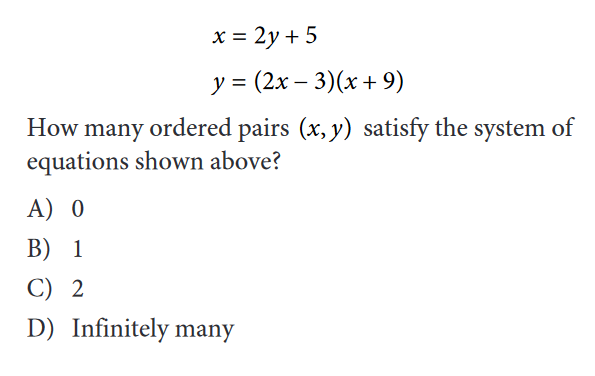Here’s how you solve it:

1. We can solve this using substitution. Substitute the second equation into the first and expand the quadratic:

$$x=2[(2x-3)(x+9)]+5$$

$$x=2(2x^2+15x-27)+5$$

$$x=4x^2+30x-49$$

2. Next, move the x from the left-hand side over to the right. The roots of this quadratic will give us the solution to the system of equations above.

$$0=4x^2+29x-49$$

3. Since the roots of the above quadratic will give us the solution to the system of equations above, we can use the discriminant of the quadratic formula to find out how many solutions there are. The discriminant is the part of the quadratic formula under the radical, or $$b^2-4ac$$. If the discriminant is positive, there are 2 solutions; if the discriminant is 0, there is 1 real solution (or a repeated solution); if the discriminant is negative, there are no real solutions. Substituting the numbers from the equation into the discriminant formula gives us $$29^2-4(4)(49)=841-784=57$$. This is a positive number, which means there are 2 solutions to the system of equations.

Question 11: Calculator permitted, grid-in response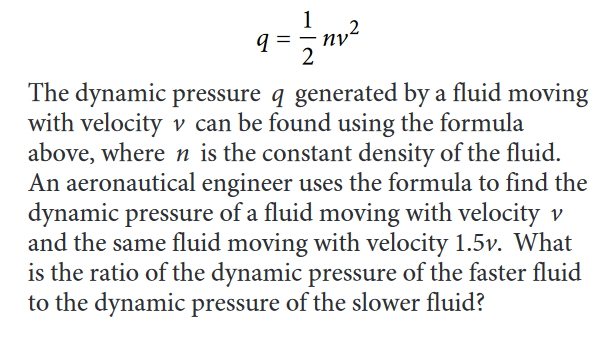Category: Passport to Advanced Math—determining effect of one variable on another

Here’s how you solve it:

1. This is similar to the earlier problem where there are only variables, but if we proceed step-by-step and manipulate the equation carefully, we can find the solution. In this problem, we have two fluids, and one of them is moving at a velocity of $$1$$ and the other at $$1.5$$. We can substitute the $$1.5$$ into the equation to see how it might affect $$q$$, or the dynamic pressure.

$$q=\frac{1}{2}n(1.5v)^2$$

2. Using exponent rules (from Question 3 above) we can rewrite $$(1.5v)^2=1.5^2v^2$$. Squaring $$1.5$$ gives us $$2.25$$.

3. For our fluid moving at a velocity of $$1.5$$, we thus have: $$q=\frac{1}{2}n(2.25)v^2$$. For our fluid moving at a velocity of $$1$$, we have $$q=\frac{1}{2}n(1)v^2$$.  We can thus see that the dynamic pressure of the faster fluid will be $$2.25$$ times that of the slower fluid.

Question 12: Calculator not-permitted, grid-in response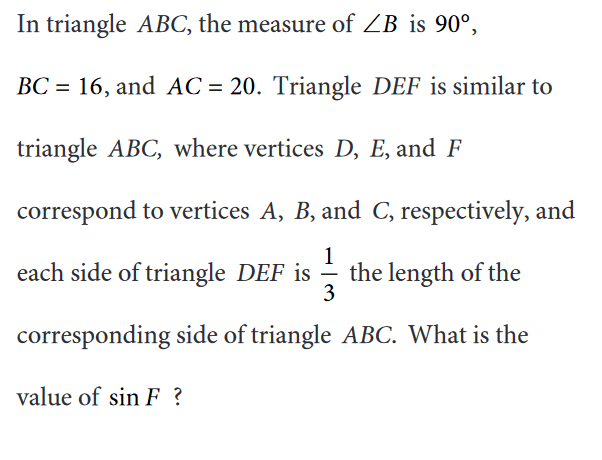Here’s how you solve it:

1. If you’re given a word problem that involves geometry or trigonometry and no diagram, you should draw yourself a diagram and label the information. This often makes it very clear how to find the answer. Here’s our diagram:2. Many students know about the 30-60-90 and the 45-45-90 right triangles, and information about these triangles is included on the first page of each SAT math portion. However, you should also be on the lookout for the 3-4-5 and 5-12-13 right triangles. College Board likes to use these triangles and their similar counterparts because they have nice, whole-number sides that make calculations easy. Knowing this, we can see that Triangle ABC is similar to the 3-4-5 triangle, each side of ABC 4 times the length of the 3-4-5 triangle.

3. Knowing that triangle ABC is similar to a 3-4-5 triangle, and that triangle DEF is also similar to a 3-4-5 triangle, we can find $$sin\:F$$ by substituting 3-4-5 for the sides of DEF and using the definition of sine: $$sin\:\theta\:=\frac{opposite}{hypotenuse}$$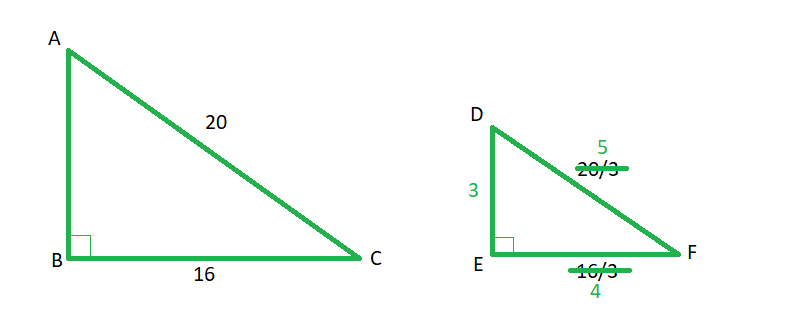4. With respect to $$F$$, the opposite side is $$3$$ and the hypotenuse is $$5$$. This means that $$sin\:F=\frac{3}{5}$$, or $$0.6$$.

Question 13: Calculator permitted, multiple choice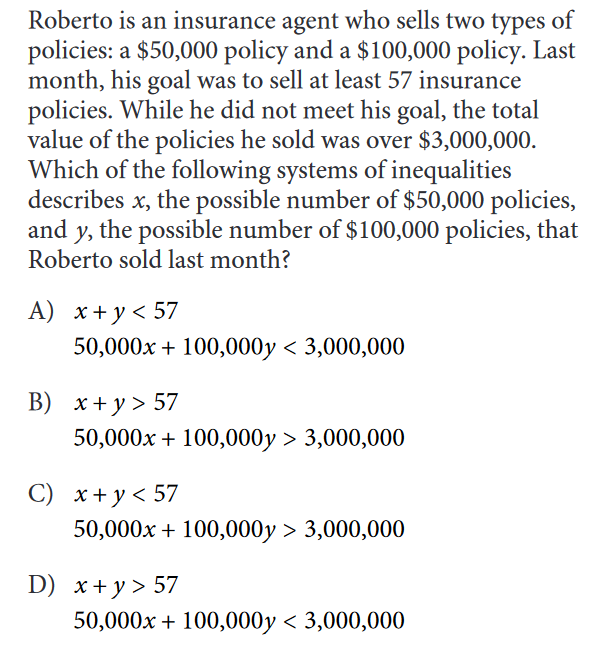Category: Heart of Algebra—linear equations and inequalities in context

Here’s how you solve it:

1. You’ll need to be able to write equations that reflect the context described in a word problem for several questions on the SAT, both linear context and non-linear contexts. If you have trouble “translating” word problems into math, this is a skill you’ll want to work on! Rewriting word problems to include words like equal to, less than, more than, sum, and so on can help you easily translate the problem into equations and inequalities.

2. We’re told that Roberto wanted to sell $$57$$ insurance policies but he didn’t meet his goal, which means that he sold less than $$57$$ insurance policies. Given that $$x$$ represents the number of $$\50,000$$ policies sold and $$y$$ represents the number of $$\100,000$$ policies sold, the sum of these two numbers is less than $$57$$. This is represented as $$x+y\:\lt\:57$$. We can eliminate choices B and D.

3. Next, we’re told that the value of all the policies sold was more than $$\3,000,000$$. We need to multiply the value of the policy by the number of that type of policy, which gives us $$50,000x+100,000y\:\gt\:3,000,000$$. Choice C is the only answer that reflects Roberto’s insurance policy sales.

Question 14: Calculator permitted, multiple choiceCategory: Problem Solving and Data Analysis—Statistics

Here’s how you solve it:

1. These kinds of problems can surprise students because there isn’t any obvious calculation or “math” to do. Instead, you’re being asked to interpret the results based on given information, and you’ll need to know statistics to correctly interpret these types of problems. In this case, you’ll want to draw upon knowledge of population parameters, random sampling, and random assignment:

• A population parameter is a numerical value describing a characteristic of a population. For example, we’re told that the population targeted by this treatment are “people with poor eyesight.” While not given a number, any conclusion we draw can only be made regarding people who fall under this parameter.
• Random sampling means that the subjects in the sample were selected at random (without bias) from the entire population in question. If random sampling is used, the results can be generalized to the entire population.
• Random assignment means that subjects in the sample were assigned to a treatment at random (without bias). If random assignment is used, it might be appropriate to make conclusions regarding cause and effect.

2. The SAT is very particular when including statistical clues and knowing the definitions of statistics. For example, if random sampling is not mentioned in a problem, then the results cannot be generalized to the entire population. Knowing the above, we can see that random sampling and random assignment are explicitly mentioned in this case. We can reasonably conclude cause and effect to be generalized to the population in question, or that it’s likely that Treatment X will improve the eyesight of people who have poor eyesight. The other choices are either too confident in their conclusion or do not specify the population in question.

Question 15: Calculator not-permitted, grid-in responseHere’s how you solve it:

1. The point $$A$$ is $$(3,1)$$. We can create a triangle to find the degree measure of $$\angle{AOB}$$. When we do this, we can use the information the SAT provides to see that this is a 30-60-90 triangle and that the hypotenuse (or the radius of the circle) is $$2$$.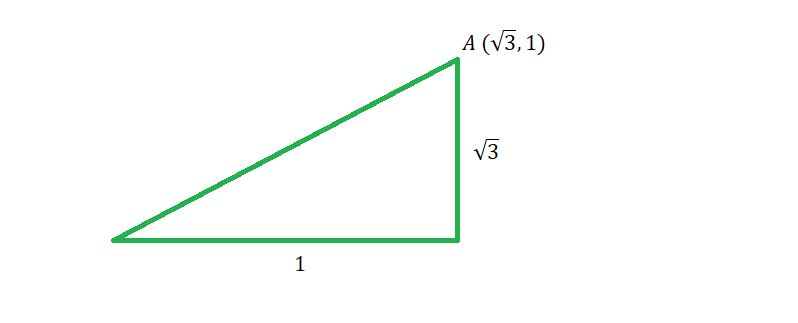2. This means that $$\angle{AOB}$$ is $$30$$ degrees. To convert from degrees to radians, we multiply by $$\frac{\pi}{180}$$. This is what we get: $$30\:\cdot\:\frac{\pi}{180}=\frac{\pi}{6}$$

3. Since $$a$$ is the denominator, the answer is $$6$$.

Final Tips

We hope that you have a good sense of the type of questions that you might see on the SAT, but just remember that these questions aren’t a complete representation of the topics you could be tested on. Review the questions above and ask yourself: which ones were the most challenging to you? Which topics do you need to brush up on?

Check out our free guide with our top 8 tips for mastering the SAT.